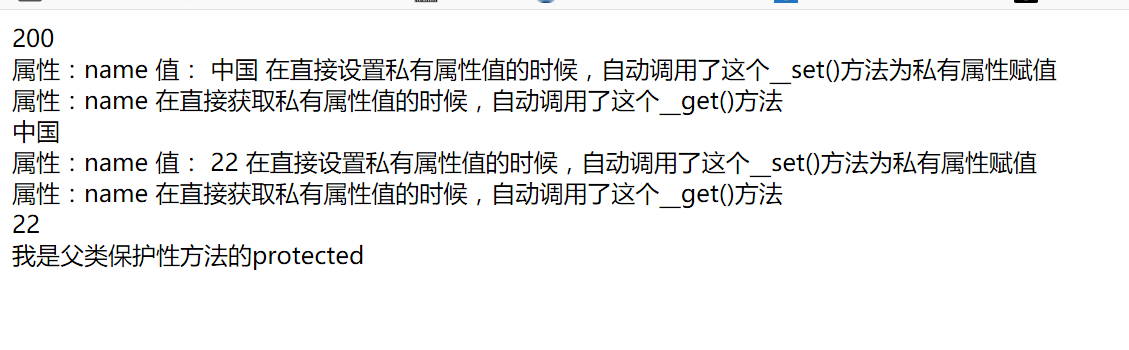# public private protected 修饰符整理

1.public定义的类或方法；任何类的实例都可以访问

2.private定义的属性和方法；只能该类内部使用；如果子类要访问父类的private属性；必须实现__set()和__get()方法；

3.protected定义的属性和方法；该本类和子类或者父类内部使用。实例对象不可以访问；

<?php
class a
{
private $c=100; private$name = 'wang san';
public $age=200; //设置私有属性 public function __set($a,$val){ echo "属性：{$a} 值： {$val} 在直接设置私有属性值的时候，自动调用了这个__set()方法为私有属性赋值<br>";$this->$a =$val;
}
//获取私有属性
public function __get($a){ echo "属性：{$a} 在直接获取私有属性值的时候，自动调用了这个__get()方法<br>";
return $this->$a;
}

private function getAge(){
echo "我是父类私有的private";
}
protected function getName(){
echo "我是父类保护性方法的protected";
}
function getALL(){
echo "我是父类public方法";
}
}

$a = new a(); echo$a->age.'<br>';
$a->name = '中国'; //必须实现了魔术方法__set 和 __get方法 实例对象才可以访问 echo$a->name.'<br>'; //输出：中国

class b extends a
{
public function getName2(){
$this->getName();//子类内部可以使用；但是外部不可以使用 } public function getAge2(){$this->getAge(); //报错 不允许子类内部使用
}
}
$b = new b();$b->name = 22;
echo $b->name.'<br>'; //echo$b->getAge(); //错误 子类对实例对象 不可以是使用
//echo $b->getName(); //错误 子类对实例对象 不可以是使用 echo$b->getName2(); //可以使用；子类对实例对象 可以内部使用
//echo \$b->getAge2(); //错误 子类对实例对象 可以内部使用posted @ 2019-05-26 15:14  王默默  阅读(141)  评论(0编辑  收藏  举报